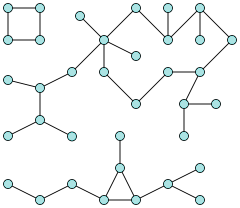# Time complexity of recursive functions [Master theorem]

yourbasic.org

It's often possible to compute the time complexity of a recursive function by formulating and solving a recurrence relation.This text contains a few examples and a formula, the “master theorem”, which gives the solution to a class of recurrence relations that often show up when analyzing recursive functions.

We also show how to analyze recursive algorithms that depend on the size and shape of a data structure.

## Recurrence relation

As an introduction we show that the following recursive function has linear time complexity.

``````// Sum returns the sum 1 + 2 + ... + n, where n >= 1.
func Sum(n int) int {
if n == 1 {
return 1
}
return n + Sum(n-1)
}
``````

Let the function T(n) denote the number of elementary operations performed by the function call `Sum(n)`.

We identify two properties of T(n).

• Since `Sum(1)` is computed using a fixed number of operations k1, T(1) = k1.
• If n > 1 the function will perform a fixed number of operations k2, and in addition, it will make a recursive call to `Sum(n-1)`. This recursive call will perform T(n-1) operations. In total, we get T(n) = k2 + T(n-1).

If we are only looking for an asymptotic estimate of the time complexity, we don’t need to specify the actual values of the constants k1 and k2. Instead, we let k1 = k2 = 1. To find the time complexity for the `Sum` function can then be reduced to solving the recurrence relation

• T(1) = 1,  (*)
• T(n) = 1 + T(n-1), when n > 1.  (**)

By repeatedly applying these relations, we can compute T(n) for any positive number n.

T(n) = (**)
1 + T(n-1) = (**)
1 + (1 + T(n-2)) = 2 + T(n-2) = (**)
2 + (1 + T(n-3)) = 3 + T(n-3) = …
k + T(n-k) = …
n - 1 + T(1) = (*)
n - 1 + 1= Θ(n)

The very same method can be used also for more complex recursive algorithms. Formulating the recurrences is straightforward, but solving them is sometimes more difficult.

Let’s try to compute the time complexity of this recursive implementation of binary search.

``````// Find returns the smallest index i at which x <= a[i].
// If there is no such index, it returns len(a).
// The slice must be sorted in ascending order.
func Find(a []int, x int) int {
switch len(a) {
case 0:
return 0
case 1:
if x <= a {
return 0
}
return 1
}
mid := 1 + (len(a)-1)/2
if x <= a[mid-1] {
return Find(a[:mid], x)
}
return mid + Find(a[mid:], x)
}
``````

We use the notation T(n) to mean the number of elementary operations performed by this algorithm in the worst case, when given a sorted slice of n elements.

Once again, we simplify the problem by only computing the asymptotic time complexity, and let all constants be 1. Then the recurrences become

• T(1) = 1,  (*)
• T(n) = 1 + T(n/2), when n > 1.  (**)

The equation (**) captures the fact that the function performs constant work (that’s the one) and a single recursive call to a slice of size n/2.

(In fact, the slice may also end up having n/2 + 1 elements. We don’t worry about that, since we’re only looking for an asymptotic estimate.)

Once again, it’s possible to find a solution by repeated substitution.

T(n) = (**)
1 + T(n/2) = (**)
1 + (1 + T(n/4)) = 2 + T(n/4) = (**)
2 + (1 + T(n/8)) = 3 + T(n/8) = ...
k + T(n/2k) = ...
log n + T(n/2log n) = log n + T(1) = (*)
log n + 1 = Θ(log n).

## Master theorem

The master theorem is a recipe that gives asymptotic estimates for a class of recurrence relations that often show up when analyzing recursive algorithms.

Let a ≥ 1 and b > 1 be constants, let f(n) be a function, and let T(n) be a function over the positive numbers defined by the recurrence

T(n) = aT(n/b) + f(n).

If f(n) = Θ(nd), where d ≥ 0, then

• T(n) = Θ(nd) if a < bd,
• T(n) = Θ(ndlog n) if a = bd,
• T(n) = Θ(nlogba) if a > bd.

We’ll skip the proof. It isn’t hard, but long. In fact, you can use repeated substitution in the same way as in the previous examples.

Let’s check that the master theorem gives the correct solution to the recurrence in the binary search example. In this case a = 1,  b = 2, and the function f(n) = 1. This implies that f(n) = Θ(n0), i.e. d = 0. We see that a = bd, and can use the second bullet point of the master theorem to conclude that

T(n) = Θ(n0log n),

which is correct.

## Analysis without recurrence

For algorithms that operate on a data structure, it’s typically not possible to find a recurrence relation. Instead, we can count the work performed for each piece of the data structure visited by the algorithm.

Consider this graph with 36 (blue) vertices and 3 disjoint connected components.Depth-first search is an algorithm that visits all edges in a graph `G` that belong to the same connected component as vertex v.

```Algorithm DFS(G, v)
return
Mark v as visited.
// Perform some operation on v.
for all neighbors x of v
DFS(G, x)
```

The time complexity of this algorithm depends of the size and structure of the graph. For example, if we start at the top left corner of our example graph, the algorithm will visit only 4 edges.

To compute the time complexity, we can use the number of calls to `DFS` as an elementary operation: the if statement and the mark operation both run in constant time, and the for loop makes a single call to DFS for each iteration.

• Before running the algorithm, all |V| vertices must be marked as not visited. This takes Θ(|V|) time.
• Let E' be the set of all edges in the connected component visited by the algorithm. The algorithm makes two calls to `DFS` for each edge {uv} in E': one time when the algorithm visits the neighbors of u, and one time when it visits the neighbors of v.

Hence, the time complexity of the algorithm is Θ(|V| + |E'|).

### Further examples

The Your basic graph article has more examples, including Dijkstra’s algorithm, of this type of analysis.

### Previous